Processing ......FreeComputerBooks.com Links to Free Computer, Mathematics, Technical Books all over the World

An Introduction to Set Theory
Top Free Machine Learning Books 🌠 - 100% Free or Open Source!
• Title An Introduction to Set Theory
• Authors William A. R. Weiss
• Publisher: CreateSpace Independent Publishing Platform (October 25, 2014)
• Paperback: N/A
• eBook: PDF, PostScript, dvi
• Language: English
• ISBN-10: 1502970597
• ISBN-13: 978-1502970596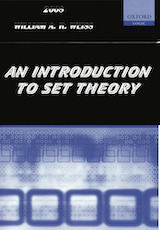Book Description

Thoroughly revised, updated, expanded, and reorganized to serve as a primary text for mathematics courses, This book covers the basics: relations, functions, orderings, finite, countable, and uncountable sets, and cardinal and ordinal numbers. It gives students sufficient grounding in a rigorous approach to the revolutionary results of set theory as well as pleasure in being able to tackle significant problems that arise from the theory.

It also provides five additional self-contained chapters, consolidates the material on real numbers into a single updated chapter affording flexibility in course design, supplies end-of-section problems, with hints, of varying degrees of difficulty, includes new material on normal forms and Goodstein sequences, and adds important recent ideas including filters, ultrafilters, closed unbounded and stationary sets, and partitions.

The proofs in this textbook are rigorous, clear, and complete, while remaining accessible to undergraduates who are new to upper-level mathematics. Exercises are included at the end of each section in a chapter, with useful suggestions for the more challenging exercises.

• N/A
Reviews, Ratings, and Recommdations: Related Book Categories: Read and Download Links:Similar Books:
•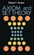Axioms and Set Theory (Robert Andre)

This book provides a very clear and well-developed approach. It covers axioms and set theory for the mathematics student on the upper undergraduate or graduate level. Throughout, emphasis is on axioms and theorems; proofs are informal.

•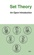Set Theory: An Open Introduction (Richard Zach)

This book is a brief introduction to the philosophy of set theory. It is written for students with a little background in logic, and some high school mathematics, aims to scratch the tip of the surface of the philosophy of set theory.

•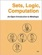Sets, Logic, Computation: An Open Introduction to Metalogic

It covers naive set theory, first-order logic, sequent calculus and natural deduction, the completeness, compactness, and Löwenheim-Skolem theorems, Turing machines, and the undecidability of the halting problem and of first-order logic.

•Set Theoretic Approach to Algebraic Structures in Mathematics

This book brings out how sets in algebraic structures can be used to construct the most generalized algebraic structures, like set linear algebra / vector space, set ideals in groups and rings and semigroups, and topological set vector spaces.

•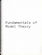Fundamentals of Model Theory (William Weiss, et al)

This book is a concluding discussion focuses on the relationship between proofs and formal derivations, and the role proofs may play as part of a general theory of evidence. It is a primer which will give someone a self contained overview of the subject.

•Introduction to Mathematical Logic (Vilnis Detlovs, et al)

This book explores the principal topics of mathematical logic. It covers propositional logic, first-order logic, first-order number theory, axiomatic set theory, and the theory of computability. Discusses the major results of Gödel, Church, Kleene, Rosser, and Turing.

•A Friendly Introduction to Mathematical Logic (Chris Leary)

In this user-friendly book, readers with no previous study in the field are introduced to the basics of model theory, proof theory, and computability theory, leading to rigorous proofs of Gödel's First and Second Incompleteness Theorems.

•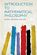Introduction to Mathematical Philosophy (Bertrand Russell)

Requiring neither prior knowledge of mathematics nor aptitude for mathematical symbolism, the book serves as essential reading for anyone interested in the intersection of mathematics and logic and in the development of analytic philosophy.

Book Categories
 :All CategoriesTop Free BooksRecent BooksMiscellaneous BooksComputer EngineeringComputer LanguagesComputer ScienceData Science/DatabasesJava and Java EE (J2EE)Linux and UnixMathematicsMicrosoft and .NETMobile ComputingNetworking and CommunicationsSoftware EngineeringSpecial TopicsWeb Programming
Other Categories